### Special Relativity

We’ve seen that simultaneous events according to one observer are not necessarily simultaneous according to another observer. The business of relativity is finding a way to express observations in one frame of reference in terms of those in another. For example, if Crater assigns the coordinates $\left(t,x\right)$ to event $P$ $($red circle in the figure below$),$ what coordinates $\left(t^\prime, x^\prime\right)$ would Alyssa assign to it?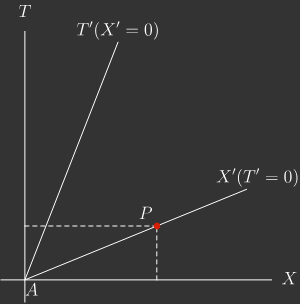On a planet where we have so-called universal time that directs flight schedules and market closing bells, the idea that time could be different for different observers seems disconnected from reality. Galilean relativity allowed observers to disagree on position coordinates, but not time coordinates. In Einsteinian relativity, we have to get used to the idea that, in general, observers agree on neither position nor time.

In this quiz, we’re going to reflect on our findings so far and use them to derive the so-called Lorentz transformation, the core machinery of special relativity which maps between coordinates in different inertial frames.

# Derive: Lorentz Transformations

To get started, let's think about the properties that the Lorentz transformation must have. In this case, we'll pretend we're Crater measuring the distance between Alyssa and Bev and using the Lorentz transformation to find the same distance according to Alyssa and Bev.

Empty space is homogeneous (that means physics is the same at every point), so there can be no special points. If we shift Crater's origin in $F,$ the distance he measures between Alyssa and Bev in $F^\prime$ shouldn't change. Put simply, in the scenario below, Crater's position should not affect the distance between Alyssa and Bev in their own frame.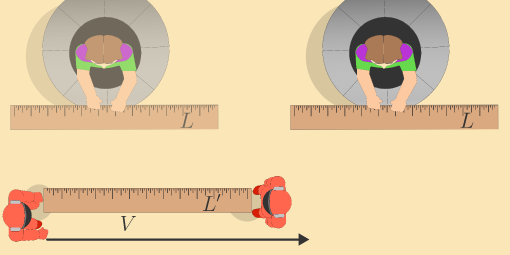In slightly more mathematical terms, we don't expect mapping under the Lorentz transformation to be affected by origin shifts such as $\overbrace{\left(t=0, x=0\right)}^\textrm{Old origin} \longrightarrow \overbrace{\left(t=0, x=+ \Delta x\right)}^\textrm{Shifted origin}.$

Which of the potential transformations for $x^\prime$ is independent of the choice of origin in frame $F?$

Assume $A, B,$ and $C$ are real numbers.

# Derive: Lorentz Transformations

In the last quiz, we learned two important features of Alyssa's reference frame that will guide us for the rest of this quiz.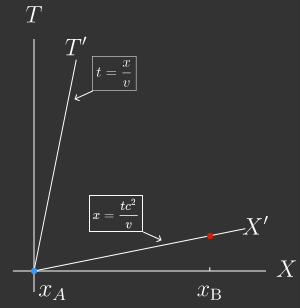1. The spatial origin of her frame moves along with her. This means that if she follows the path $x=vt$ in Crater's frame $F,$ the Lorentz transformation has to map her position to $x^\prime=0$ in her frame $F^\prime.$
2. Any events that lie along the line $t=vx/c^2$ in frame $F$ are simultaneous in $F^\prime.$ This means that the Lorentz transformation has to map any point along that line in $F$ to $t^\prime=0$ in $F^\prime.$

# Derive: Lorentz Transformations

We now have a general constraint for the form of the Lorentz transformation: it needs to be linear in space $x$ and time $t$.

To make progress from here, we have to reason physically. One of our lessons from the last quiz is that an observer is always at the origin of their own reference frame. It follows that the Lorentz transformation must abide this.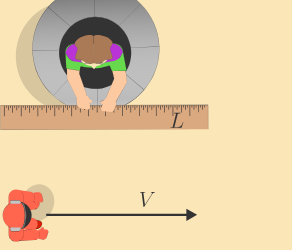Suppose Alyssa moves in a straight line so that Crater measures her position as $x = vt$ in $F.$ What is Alyssa's position in her own frame? In other words, what position $x^\prime$ in $F^\prime$ does $x = vt$ need to map to?

# Derive: Lorentz Transformations

As we've seen, the transformation $x^\prime = f(x, t, v)$ has to yield zero when $x = vt.$ Since $f$ also needs be linear, $f$ must be proportional to $\left(x-vt\right):$ $\boxed{x^\prime \propto (x - vt)}.$ We've also seen that all points along the line $t =vx/c^2$ in $F$ are simultaneous with the origin in the moving frame $F^\prime,$ so the transformation $t^\prime(x,t)$ has to map points that satisfy $t = vx/c^2$ to $t^\prime = 0.$

As with the spatial transformation above, this means that $t^\prime$ must be proportional to $\big(t - vx/c^2\big):$ $\boxed{t^\prime \propto \left(t - \frac {vx}{c^2}\right)}.$

# Derive: Lorentz Transformations

As it turns out, $x^\prime = \left(x-vt\right)$ and $t^\prime = \left(t - vx/c^2\right)$ are not the most general forms the transformations for $x^\prime$ and $t^\prime$ can take while satisfying our demands.

Remember, we want

1. for the transformations to be independent of where we place the origin, and
2. for the transformations to be symmetric between the two frames (i.e. the second postulate of relativity).

As long as $x^\prime$ and $t^\prime$ are linear functions of $x$ and $t,$ the first condition will be met.

What kind of function could we multiply $\left(x-vt\right)$ and $\left(t - vx/c^2\right)$ by and still satisfy the second condition?

# Derive: Lorentz Transformations

Given no preferred direction, the general forms are \begin{aligned} x^\prime &= (x - vt) \cdot \gamma\big(v^2\big) \\ t^\prime &= \left(t - \frac{vx}{c^2}\right) \cdot \phi\big(v^2\big). \end{aligned}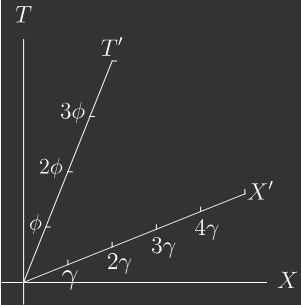What are the functions $\gamma$ and $\phi?$ We'll deal with their exact form next, but to help us along the way, we can find a relationship between them. Einstein's first postulate asserts that the speed of light is constant across all frames of reference, i.e. that $x = ct$ in all frames. If we transform to another frame of reference, it must be the case that $x^\prime = ct^\prime.$

Use this observation to solve for $\gamma\big(v^2\big)$ in terms of $\phi\big(v^2\big).$

Hint: use the Lorentz transformations to find $\dfrac{x^\prime}{t^\prime}$ when $x = ct.$

# Derive: Lorentz Transformations

Up to here, we've used the isotropy of empty space (the fact that it's the same in all directions), and the preservation of the origin to chisel down the mathematical form of the Lorentz transformation.

All that remains is to find the expression for the function $\gamma\big(v^2\big):$ \begin{aligned} x^\prime &= \left(x - vt\right) \gamma\big(v^2\big) \\ t^\prime &= \left(t - \dfrac{vx}{c^2}\right) \gamma\big(v^2\big). \end{aligned}

# Derive: Lorentz Transformations

Einstein's second postulate asserts that there is no frame that has privilege over another. This means that the Lorentz transformation needs to be symmetric between frames.

If we write $x^\prime$ and $t^\prime$ in terms of $x$ and $t,$ then we should be able to write the same equations for $x$ and $t$ in terms of $x^\prime$ and $t^\prime,$ with the sole difference that $x^\prime$ moves with speed $-v$ relative to $x.$

In other words, it must be true that \boxed{\begin{aligned} x &= (x^\prime + vt^\prime)\gamma\big(v^2\big) \\ t &= \left(t^\prime + \frac{vx^\prime}{c^2}\right)\gamma\big(v^2\big). \end{aligned}} Eliminate $x$ and $t$ from the expression for $x^\prime$ and solve for $\gamma\big(v^2\big).$

Hint: Plug the expressions for Lorentz transformation for $x$ and $t$ into the Lorentz transformation for $x^\prime$; you'll find an equation in terms of $v$ and $c.$

# Derive: Lorentz Transformations

At last, we have the Lorentz transformations between space and time: \begin{aligned} x^\prime &= \dfrac{x - vt}{\sqrt{1 - \dfrac{v^2}{c^2}}} \\\\ t^\prime &= \dfrac{t - vx/c^2}{\sqrt{1 - \dfrac{v^2}{c^2}}}. \end{aligned} After all that work, the transformations have a simple interpretation. If we see another observer moving along our $X$-axis, the lines representing events simultaneous to them are bent up from our $x$-axis $($this is the $x=vt$ piece$)$ and the lines representing events that are colocated to them are bent down from our $t$-axis $\big($this is the $t = vx/c^2$ piece$\big).$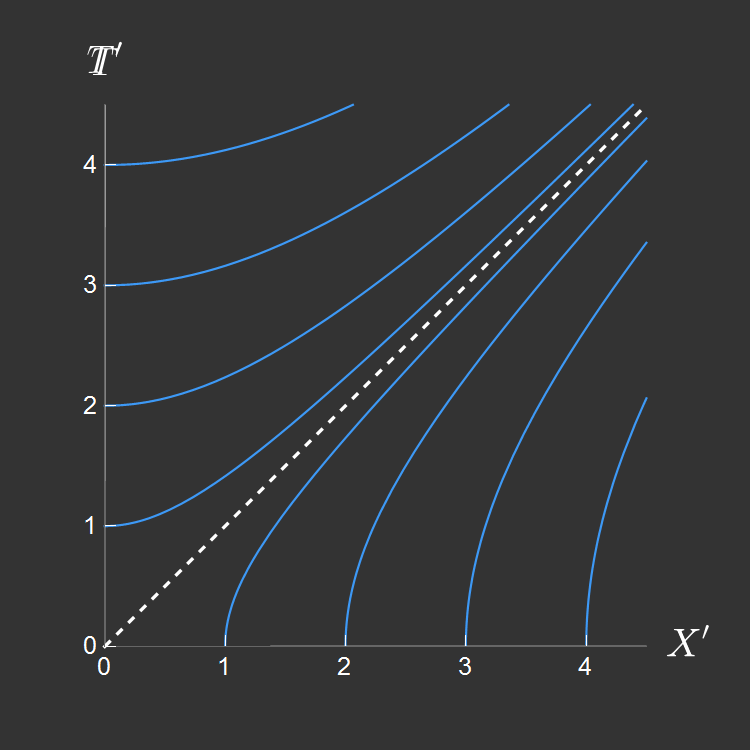If we apply this correction to the spacetime grid, we can plot contours of points with equal $x^\prime$- and $t^\prime$-coordinates on top of the $x, t$ frame and see how length and time scale around the space.

In sum, as the relative velocity between $F$ and $F^\prime$ increases, the primed axes bend toward the worldline of a light beam and the tickmarks spread toward infinite separation (quantitatively, the ticks along the primed axes are inflated by the factor $1/\sqrt{1-v^2/c^2}$ relative to the unprimed ticks). We will see in the next chapter that we can think of a Lorentz transformation as a rotation of time and space into one another.

# Derive: Lorentz Transformations

Before we leave, we need to address the coordinates missing from the room: $y^\prime$ and $z^\prime$! To answer this, let's consider a thought experiment involving our favorite painters, Bev and Alyssa.Suppose Bev stands holding a paintbrush at face level, hoping to paint Alyssa's face with a blue stripe. Likewise, Alyssa (who travels with speed $v$ along the $x$-axis) holds her paintbrush at face level, hoping to paint Bev's face with a red stripe.

Where does the blue stripe end up on Alyssa?

# Derive: Lorentz Transformations

×